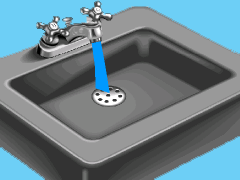Ch 7. Incompressible and Inviscid Flow Multimedia Engineering Fluids Bernoulli'sEquation FlowMeasurements Superpositionof Flows Flow aroundCylinder
 Chapter 1. Basics 2. Fluid Statics 3. Kinematics 4. Laws (Integral) 5. Laws (Diff.) 6. Modeling/Similitude 7. Inviscid 8. Viscous 9. External Flow 10. Open-Channel Appendix Basic Math Units Basic Equations Water/Air Tables Sections Search eBooks Dynamics Fluids Math Mechanics Statics Thermodynamics Author(s): Chean Chin Ngo Kurt Gramoll ©Kurt GramollFLUID MECHANICS - CASE STUDY IntroductionProblem Graphic Upon returning from a one-month trip to Europe, Bob shockingly discovers he left the water running from the tap the whole time that he was gone. Using a rotameter, he would like to find out the water flow rate, determine how much water is wasted, and what is the cost associated with it. The float of the rotameter is 10 mm from its base. Questions Determine the water flow rate based on the position of the float. Find the total volume and cost of the water wasted. Approach The float of the rotameter is a 316 stainless steel ball with a radius of 3 mm and the density of the ball is 8,040 kg/m3. Assume the drag coefficient is 0.5 and the density of the water is 1000 kg/m3. The area of the tapered tube is A = (π/4) (6 + 0.15y) mm2 The cost of the water is \$1.75/1000 gals. Assume Bob was gone for 30 days.

Practice Homework and Test problems now available in the 'Eng Fluids' mobile app
Includes over 250 problems with complete detailed solutions.
Available now at the Google Play Store and Apple App Store.